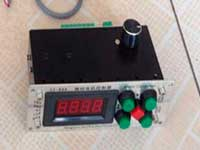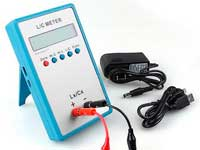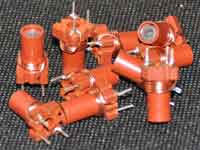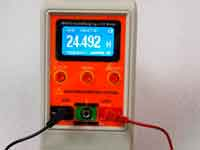About LC-circuit for beginners - 3.5 out of 5 based on 2 votes
Rating 3.50 (2 Votes)

About an oscillator circuit for beginnersFor beginning radio-amateurs it would be desirable to give a few information about parameters of LC oscillator circuit. After all coil inductance is the base of it. An oscillator circuit, as it is known, consists from coil inductance and condenser.

The main characteristics of a LC oscillator circuit are:• Resonance frequency
• Q - factor
• Equivalent resistance
• Bandpass range

Scheme1.

Resonance frequency of LC circuit calculates by formula:Where L and C in Henry and Farads accordingly

However, it is necessary to remember one very much an important point. "C" is not nominal capacitance of the condenser of a LC circuit. It consists of the many capacitances - this condenser, stray capacitance of the coil, capacitance of the external circuits connected to a LC circuit (for example output and input capacitances of a transistor), mounting stray capacitance. These introduced capacitances are essential, especially on high frequencies where they are commensurable with capacitance of the LC circuit condenser. They are necessary for considering! Sometimes at forums it is possible to read any messages: "I calculated the coil, using such program, made a homebrew coil, and then it appeared that coil should be making by a method " manual selecting of turns ", so this program is bad ". The program calculates correctly. Really, it calculates the coil in the unloaded LC circuit, but you must taking into account the design features.

Theoretically, all aforesaid refer to inductance "L" , however in the realities introduced in a circuit of inductances 10 times less and them in most cases it is possible not to consider.

Q-factor of a unloaded LC circuit Q is defined by Q-factor of the coil QL and the condenser QC. QL depends on resistance rL (see scheme1.), equivalent to losses of electrical energy in a wire, in wire insulation, a coil-former, sheet, core of the coil. QL = 2πƒ L / rL. Normally depending on quality design of the coil inductance and on quality applied materials – QL≈50÷250.

Q-factor of condenser QC depends on resistance RC, equivalent to losses electrical energy in condenser. QC = 2πƒRC. Usually QC≈400÷1000.

Various resistance of losses (rL,RC) it is possible, for convenience of calculations to replace with one resistance Re, connected in parallel to an ideal LC circuit lost-free which is called as the equivalent resistance of a circuit. It characterizes all losses of a real circuit and is equal to resistance of a circuit on resonance frequency. Note, that on resonance frequency reactive impedances of the coil inductance and the condenser are equal and opposite on a sign and compensate each other, as a result the general impedance of a circuit purely actively.

Value Re is connected to other parameters of a circuit following ratios: Re = 2πƒLQ = 2πƒCQ

Here again there is an important point. The additional resistance that imported from external circuits are connected to a LC circuit parallels to Re. Thus Re and hence Q are decreasing. And for high-Q LC circuits, this reduction can be essential. To minimize influence of exterior circuits on a contour, apply the partial switching-on through a capacity divider, coil tap, or apply the communication coil.

The bandwidth is equal to frequency band where value of circuit transmissions it is equal 70,7% from transfer efficiency on resonance frequency.The ratio is valid: Q = f/Δf , which can be used for measurement of Q-factor of a real circuit.

Summing up, I will mark that the oscillator circuit is widely used in radio engineering devices for a filtration of electrical oscillations, for phase turn, for the matching of impedance and for other purposes. At LC circuit calculation it is is necessary to consider parameters of the external circuits connected to the LC circuit and qualitative characteristics of LC circuit components, especially inductance coil.

Сomments from anonymous guests are enabled with moderation.

Coil Winding MachineUS \$187.53
The system consists of individual components including a controller, a stepping motor, a foot switch, power adapter and output shaft. This design makes it very easy for you to integrate the system into your own project.

LC Meter LC200AUS \$37.99
Measuring Capacitance Range:
0.01 pF - 100mF
Measuring Inductance Range:
0.001 uH - 100H
Measurement accuracy:1%.

RF InductorsUS \$6.43
Variable Ferrite Core Inductor coil set 3.5Turns 25-100MHZ

Auto Ranging LCR MeterUS \$63.07
Meter can measure 0.00pF to 100.00mF capacitance, 0.000uH to 100.00H inductance and 0.00? to 10.000M? resistance. Very suitable for small value capacitor and inductor measurement.